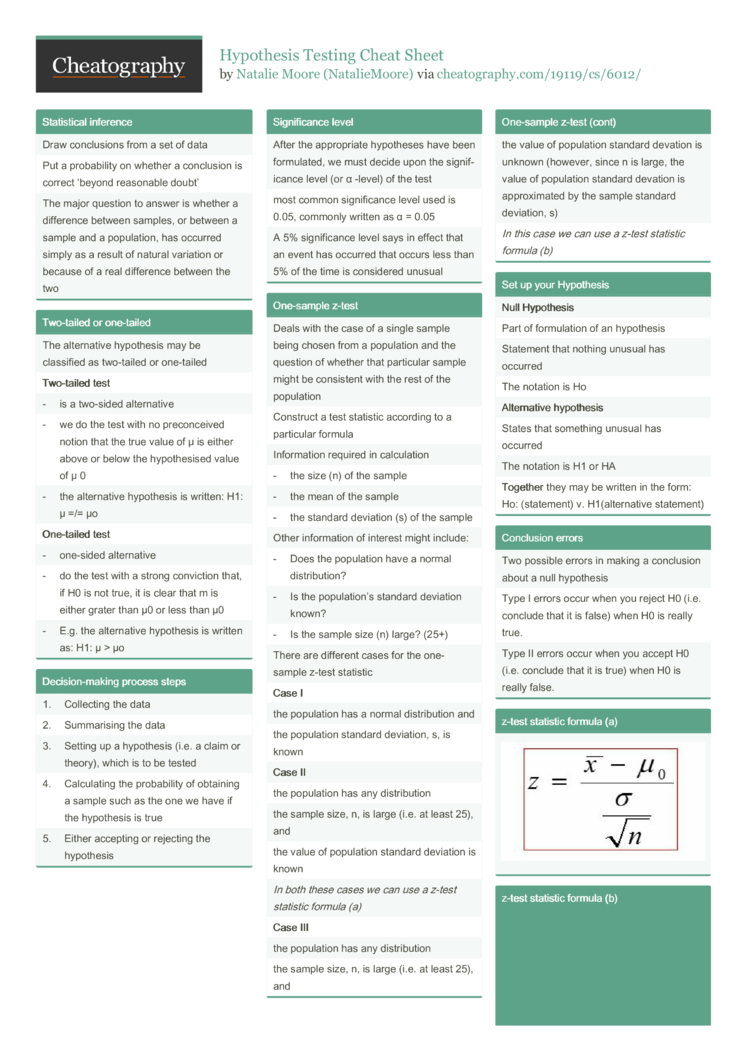# Hypothesis Testing Cheat Sheet by NatalieMoore

### Statis­tical inference

 Draw conclu­sions from a set of data Put a probab­ility on whether a conclusion is correct ‘beyond reasonable doubt’ The major question to answer is whether a difference between samples, or between a sample and a popula­tion, has occurred simply as a result of natural variation or because of a real difference between the two

### Two-tailed or one-tailed

 The altern­ative hypothesis may be classified as two-tailed or one-tailed Two-­tailed test - is a two-sided altern­ative - we do the test with no precon­ceived notion that the true value of μ is either above or below the hypoth­esised value of μ 0 - the altern­ative hypothesis is written: H1: µ =/= µo One-­tailed test - one-sided altern­ative - do the test with a strong conviction that, if H0 is not true, it is clear that m is either grater than µ0 or less than µ0 - E.g. the altern­ative hypothesis is written as: H1: µ > µo

### Decisi­on-­making process steps

 1 Collecting the data 2 Summar­ising the data 3 Setting up a hypothesis (i.e. a claim or theory), which is to be tested 4 Calcul­ating the probab­ility of obtaining a sample such as the one we have if the hypothesis is true 5 Either accepting or rejecting the hypothesis

### Signif­icance level

 After the approp­riate hypotheses have been formul­ated, we must decide upon the signif­icance level (or α -level) of the test most common signif­icance level used is 0.05, commonly written as α = 0.05 A 5% signif­icance level says in effect that an event has occurred that occurs less than 5% of the time is considered unusual

### One-sample z-test

 Deals with the case of a single sample being chosen from a population and the question of whether that particular sample might be consistent with the rest of the population Construct a test statistic according to a particular formula Inform­ation required in calcul­ation - the size (n) of the sample - the mean of the sample - the standard deviation (s) of the sample Other inform­ation of interest might include: - Does the population have a normal distri­bution? - Is the popula­tion’s standard deviation known? - Is the sample size (n) large? (25+) There are different cases for the one-sample z-test statistic Case I the population has a normal distri­bution and the population standard deviation, s, is known Case II the population has any distri­bution the sample size, n, is large (i.e. at least 25), and the value of population standard deviation is known In both these cases we can use a z-test statistic formula (a) Case III the population has any distri­bution the sample size, n, is large (i.e. at least 25), and the value of population standard devation is unknown (however, since n is large, the value of population standard devation is approx­imated by the sample standard deviation, s) In this case we can use a z-test statistic formula (b)

 Null Hypoth­esis Part of formul­ation of an hypothesis Statement that nothing unusual has occurred The notation is Ho Alte­rnative hypothesis States that something unusual has occurred The notation is H1 or HA Toge­ther they may be written in the form: Ho: (state­ment) v. H1(alt­ern­ative statement)

### Conclusion errors

 Two possible errors in making a conclusion about a null hypothesis Type I errors occur when you reject H0 (i.e. conclude that it is false) when H0 is really true. Type II errors occur when you accept H0 (i.e. conclude that it is true) when H0 is really false.

### z-test statistic formula (a)### z-test statistic formula (b)2 Pages
//media.cheatography.com/storage/thumb/nataliemoore_hypothesis-testing.750.jpg

PDF (recommended)